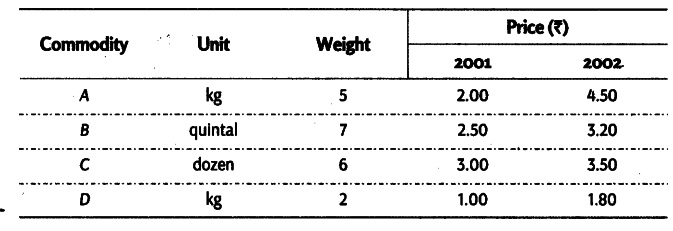# The price quotation of different commodities for 2001 and 2002 are given below

The price quotation of different commodities for 2001 and 2002 are given below. Calculate the index number for 2002 with 2001 as base year by using
(i) Simple Average of Price Relative
(ii) Weighted Average of Price Relative